# Induction motor locked rotor test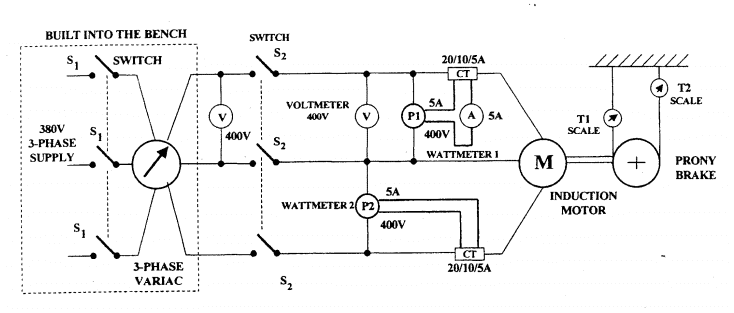For the locked rotor test I have these values,
P1 = 620W
Stator line voltage = 32V
Stator line current = 8.4A

To calculate active power/phase,

Po = (P1+P2)/3

But I don't have P2, Is P1 maybe equal to P2 ?

i doubt it - that only happens at power factor 1.0

These show why.

Is this a lab ? Using those links you could calculate what P2 should read , then go back to the bench and see how close you came.

Yes, it is

To calculate P2, I got this formula from the second link
Wa = VL*IL*cos(30+∅)
Wb = VL*IL*cos(30-∅)

If this is the formula and I know Wa = 620W then what is VL and IL

Is that how I calculate P2 ?

jim hardy
Gold Member
Dearly Missed
Good - you went to second link which is same connection as you showed .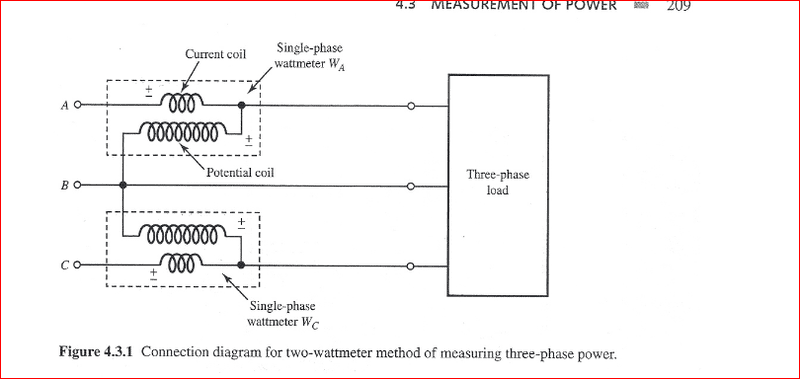then what is VL and IL

What you measured on the voltmeter and ammeter.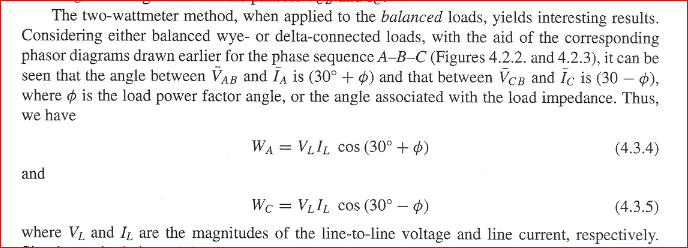Since you only measured one phase you'll have to assume it's balanced . Would be advisable to check that too when you re-test.

Observe how thorough that author was in defining his quantities
he clarified that VL means line to line not line to neutral

That is an important habit to form early ; without it three phase will be confusing.

What you measured on the voltmeter and ammeter.

If I use VL = 32V and IL = 8.4A and Wa = 620W

620 = 32*8.4*cos(30+∅)
cos(30+∅) = 2.31

But that cant be true

By the way, what I am actually trying to calculate is r1+r2' and X, given by this equivalent circuit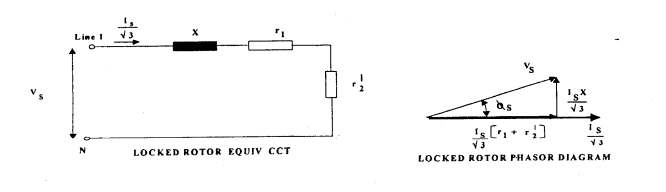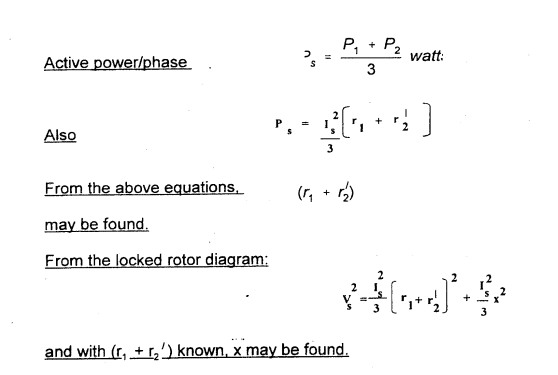jim hardy
Gold Member
Dearly Missed
If I use VL = 32V and IL = 8.4A and Wa = 620W

620 = 32*8.4*cos(30+∅)
cos(30+∅) = 2.31

But that cant be true

Good one ! I missed that, admittedly i didn't try out your numbers....

So we have an impossible result , a wattmeter reporting power factor over unity (the free energy crowd will love that !)
Therefore we must question our observations and root out what mistake(s) led us into that impossible conclusion ?

P1 reported 620 watts with 32 volts and 8.4 amps - something looks fishy there.
I note you show a CT but no PT(voltage stepdown transformer)
Look at the back of PI . Does it assume any PT ratio and CT ratios?
or Is full scale on P1 equal to 5A X 400V = 2000 Watts ?

If the latter, P1 will under-report power by the CT ratio
With CT set on 10::5 , 1240 watts would report as 620
with CT set on 20::5 , it'd take 2480 to report as 620

Sorry to be so uncertain - please realize i wasn't there and haven't seen your instruments. I'm accustomed to analog antiques in oak boxes..

In school labs it's not unusual for CT's to get blown........ they're easily hurt by passing primary current with secondary open circuited.. That pierces insulation on secondary winding and they thereafter report way low. i hope that's not your trouble.

First i'd see if you get more reasonable results from multiplying wattmeter readings by whatever was your CT ratio , 2 or 4.

old jim

P1 reported 620 watts with 32 volts and 8.4 amps - something looks fishy there.
Yeah, I think the wattmeter reading might be wrong. Will have to check it up in the lab again.
Thanks

jim hardy
Gold Member
Dearly Missed
Yeah, I think the wattmeter reading might be wrong. Will have to check it up in the lab again.
Thanks

i didnt do anything, but thanks for the feedback.

8.4 amps is the current measured where ? If on your 5 amp ammeter, the CT is being overworked and likely is introducing undue phase shift.... and so is the wattmeter's current coil..

Labs teach us more through serendipity than through the intended lesson.
Like Thoreau's Bean Field.

old jim

i didnt do anything, but thanks for the feedback.

8.4 amps is the current measured where ? If on your 5 amp ammeter, the CT is being overworked and likely is introducing undue phase shift.... and so is the wattmeter's current coil..

Labs teach us more through serendipity than through the intended lesson.
Like Thoreau's Bean Field.

old jim

The actual current measured on the ammeter was 4.2A, but the CT ratio is 2:1 so 4.2A*2 = 8.4A

jim hardy
Gold Member
Dearly Missed
The actual current measured on the ammeter was 4.2A, but the CT ratio is 2:1 so 4.2A*2 = 8.4A

That's Good news... Thanks

I know it seemed a dumb thing to ask
but i've fixed a lot of troubles by checking the dumb things first.
When working alone , one doesn't have to confess that he looked at them.
Please do not think i am talking down to you - this is just how i work. It's okay to be slow but sure footed .

So mr Wattmeter reported 620 watts with 4.2 amps and 32 volts, when he couldn't have received more than 134.4.
High by at least 4.6 , nearest integer to that is 5.

I'm guessing that Mr Wattmeter is expecting a CT with higher ratio.
But that's just a guess.
Check his nameplate, meter face, instruction leaflet ......